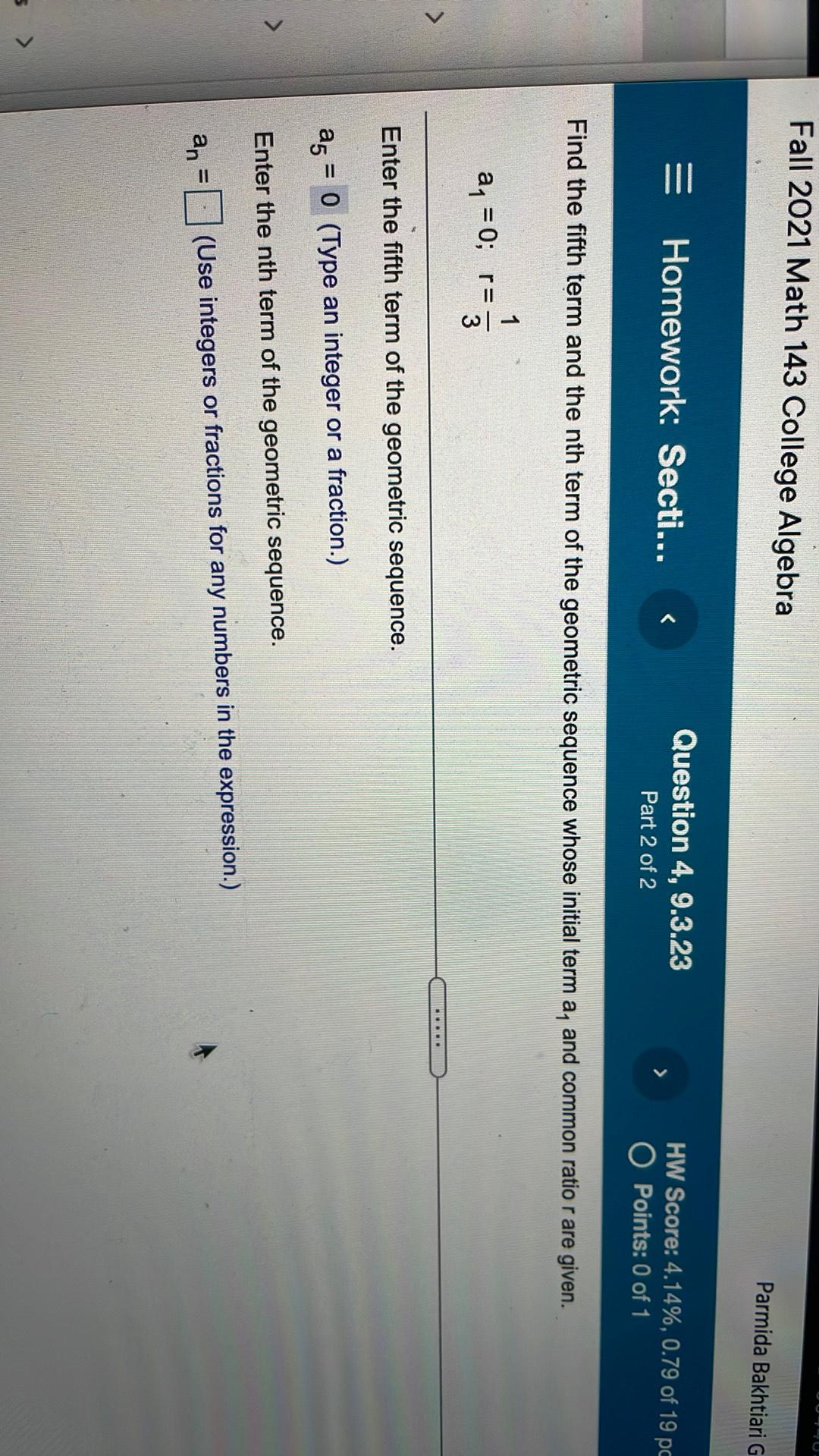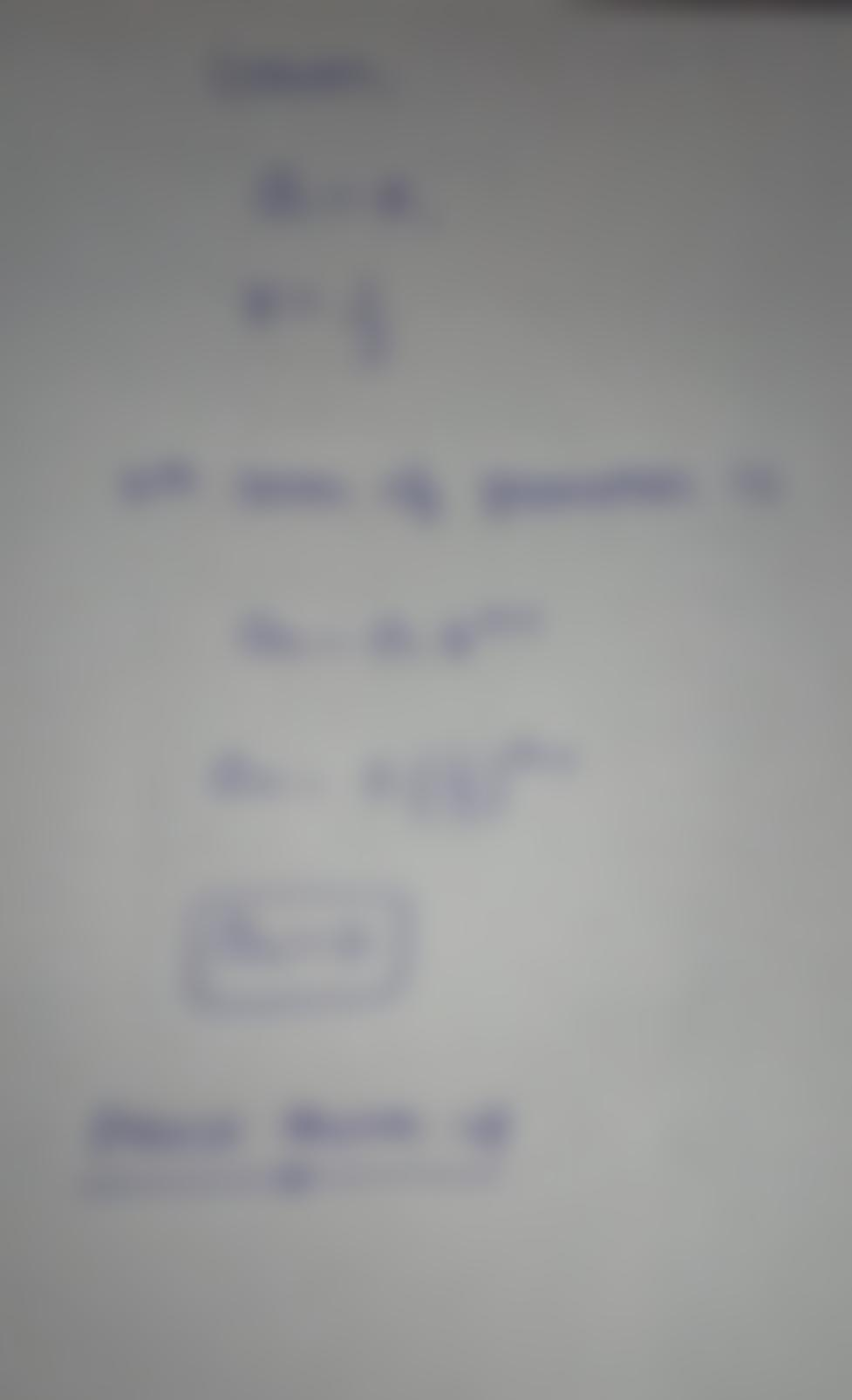Question:

Fall 2021 Math 143 College Algebra Parmida Bakhtiari G = Homework: Secti... < Question 4, 9.3.23 Part 2 of 2 > HW Score: 4.14%,Fall 2021 Math 143 College Algebra Parmida Bakhtiari G = Homework: Secti... < Question 4, 9.3.23 Part 2 of 2 > HW Score: 4.14%, 0.79 of 19 pc O Points: 0 of 1 Find the fifth term and the nth term of the geometric sequence whose initial term a, and common ratio r are given. 1 ay = 0; r= 3 > ... Enter the fifth term of the geometric sequence. = a5 = 0 (Type an integer or a fraction.) Enter the nth term of the geometric sequence. = an (Use integers or fractions for any numbers in the expression.)